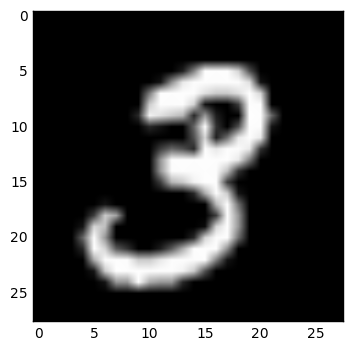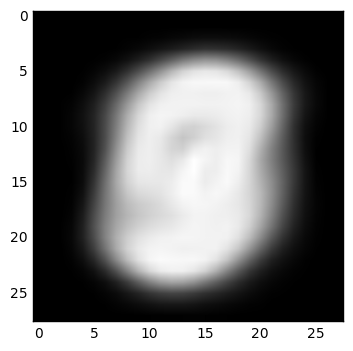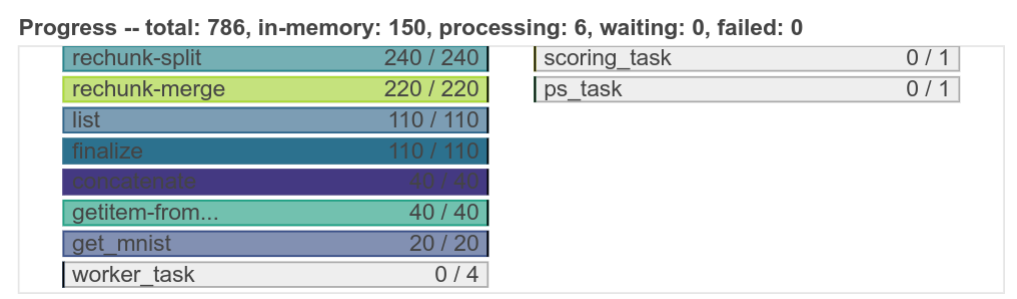This work is supported by Continuum Analytics the XDATA Program and the Data Driven Discovery Initiative from the Moore Foundation

## Summary

This post briefly describes potential interactions between Dask and TensorFlow and then goes through a concrete example using them together for distributed training with a moderately complex architecture.

This post was written in haste and the attached experiment is of low quality, see disclaimers below. A similar and much better example with XGBoost is included in the comments at the end.

## Introduction

Dask and TensorFlow both provide distributed computing in Python. TensorFlow excels at deep learning applications while Dask is more generic. We can combine both together in a few applications:

1. Simple data parallelism: hyper-parameter searches during training and predicting already-trained models against large datasets are both trivial to distribute with Dask as they would be trivial to distribute with any distributed computing system (Hadoop/Spark/Flink/etc..) We won’t discuss this topic much. It should be straightforward.
2. Deployment: A common pain point with TensorFlow is that setup isn’t well automated. This plagues all distributed systems, especially those that are run on a wide variety of cluster managers (see cluster deployment blogpost for more information). Fortunately, if you already have a Dask cluster running it’s trivial to stand up a distributed TensorFlow network on top of it running within the same processes.
3. Pre-processing: We pre-process data with dask.dataframe or dask.array, and then hand that data off to TensorFlow for training. If Dask and TensorFlow are co-located on the same processes then this movement is efficient. Working together we can build efficient and general use deep learning pipelines.

In this blogpost we look very briefly at the first case of simple parallelism. Then go into more depth on an experiment that uses Dask and TensorFlow in a more complex situation. We’ll find we can accomplish a fairly sophisticated workflow easily, both due to how sensible TensorFlow is to set up and how flexible Dask can be in advanced situations.

## Motivation and Disclaimers

Distributed deep learning is fundamentally changing the way humanity solves some very hard computing problems like natural language translation, speech-to-text transcription, image recognition, etc.. However, distributed deep learning also suffers from public excitement, which may distort our image of its utility. Distributed deep learning is not always the correct choice for most problems. This is for two reasons:

1. Focusing on single machine computation is often a better use of time. Model design, GPU hardware, etc. can have a more dramatic impact than scaling out. For newcomers to deep learning, watching online video lecture series may be a better use of time than reading this blogpost.
2. Traditional machine learning techniques like logistic regression, and gradient boosted trees can be more effective than deep learning if you have finite data. They can also sometimes provide valuable interpretability results.

Regardless, there are some concrete take-aways, even if distributed deep learning is not relevant to your application:

1. TensorFlow is straightforward to set up from Python
2. Dask is sufficiently flexible out of the box to support complex settings and workflows
3. We’ll see an example of a typical distributed learning approach that generalizes beyond deep learning.

Additionally the author does not claim expertise in deep learning and wrote this blogpost in haste.

## Simple Parallelism

Most parallel computing is simple. We easily apply one function to lots of data, perhaps with slight variation. In the case of deep learning this can enable a couple of common workflows:

1. Build many different models, train each on the same data, choose the best performing one. Using dask’s concurrent.futures interface, this looks something like the following:

# Hyperparameter search
scores = client.map(train_and_evaluate, hyper_param_list, data=data)
best = client.submit(max, scores)
best.result()

2. Given an already-trained model, use it to predict outcomes on lots of data. Here we use a big data collection like dask.dataframe:

# Distributed prediction

... # do some preprocessing here
df['outcome'] = df.map_partitions(predict)


These techniques are relatively straightforward if you have modest exposure to Dask and TensorFlow (or any other machine learning library like scikit-learn), so I’m going to ignore them for now and focus on more complex situations.

Interested readers may find this blogpost on TensorFlow and Spark of interest. It is a nice writeup that goes over these two techniques in more detail.

## A Distributed TensorFlow Application

We’re going to replicate this TensorFlow example which uses multiple machines to train a model that fits in memory using parameter servers for coordination. Our TensorFlow network will have three different kinds of servers:1. Workers: which will get updated parameters, consume training data, and use that data to generate updates to send back to the parameter servers
2. Parameter Servers: which will hold onto model parameters, synchronizing with the workers as necessary
3. Scorer: which will periodically test the current parameters against validation/test data and emit a current cross_entropy score to see how well the system is running.

This is a fairly typical approach when the model can fit in one machine, but when we want to use multiple machines to accelerate training or because data volumes are too large.

We’ll use TensorFlow to do all of the actual training and scoring. We’ll use Dask to do everything else. In particular, we’re about to do the following:

2. Set up TensorFlow workers as long-running tasks
3. Feed data from Dask to TensorFlow while scores remain poor
4. Let TensorFlow handle training using its own network

For this toy example we’re just going to use the mnist data that comes with TensorFlow. However, we’ll artificially inflate this data by concatenating it to itself many times across a cluster:

def get_mnist():
from tensorflow.examples.tutorials.mnist import input_data
return mnist.train.images, mnist.train.labels

datasets = [delayed(get_mnist)() for i in range(20)]  # 20 versions of same dataset
images = [d for d in datasets]
labels = [d for d in datasets]

images = [da.from_delayed(im, shape=(55000, 784), dtype='float32') for im in images]
labels = [da.from_delayed(la, shape=(55000, 10), dtype='float32') for la in labels]

images = da.concatenate(images, axis=0)
labels = da.concatenate(labels, axis=0)

>>> images
dask.array<concate..., shape=(1100000, 784), dtype=float32, chunksize=(55000, 784)>

images, labels = c.persist([images, labels])  # persist data in memory


This gives us a moderately large distributed array of around a million tiny images. If we wanted to we could inspect or clean up this data using normal dask.array constructs:

im = images.compute().reshape((28, 28))
plt.imshow(im, cmap='gray')im = images.mean(axis=0).compute().reshape((28, 28))
plt.imshow(im, cmap='gray')im = images.var(axis=0).compute().reshape((28, 28))
plt.imshow(im, cmap='gray')This shows off how one can use Dask collections to clean up and provide pre-processing and feature generation on data in parallel before sending it to TensorFlow. In our simple case we won’t actually do any of this, but it’s useful in more real-world situations.

Finally, after doing our preprocessing on the distributed array of all of our data we’re going to collect images and labels together and batch them into smaller chunks. Again we use some dask.array constructs and dask.delayed when things get messy.

images = images.rechunk((10000, 784))
labels = labels.rechunk((10000, 10))

images = images.to_delayed().flatten().tolist()
labels = labels.to_delayed().flatten().tolist()
batches = [delayed([im, la]) for im, la in zip(images, labels)]

batches = c.compute(batches)


Now we have a few hundred pairs of NumPy arrays in distributed memory waiting to be sent to a TensorFlow worker.

## Setting up TensorFlow workers alongside Dask workers

Dask workers are just normal Python processes. TensorFlow can launch itself from a normal Python process. We’ve made a small function here that launches TensorFlow servers alongside Dask workers using Dask’s ability to run long-running tasks and maintain user-defined state. All together, this is about 80 lines of code (including comments and docstrings) and allows us to define our TensorFlow network on top of Dask as follows:

pip install git+https://github.com/mrocklin/dask-tensorflow

from dask.distibuted import Client  # we already had this above

tf_spec, dask_spec = start_tensorflow(client, ps=1, worker=4, scorer=1)

>>> tf_spec.as_dict()
{'ps': ['192.168.100.1:2227'],
'scorer': ['192.168.100.2:2222'],
'worker': ['192.168.100.3:2223',
'192.168.100.4:2224',
'192.168.100.5:2225',
'192.168.100.6:2226']}

{'ps': ['tcp://192.168.100.1:34471'],
'scorer': ['tcp://192.168.100.2:40623'],
'worker': ['tcp://192.168.100.3:33075',
'tcp://192.168.100.4:37123',
'tcp://192.168.100.5:32839',
'tcp://192.168.100.6:36822']}


This starts three groups of TensorFlow servers in the Dask worker processes. TensorFlow will manage its own communication but co-exist right alongside Dask in the same machines and in the same shared memory spaces (note that in the specs above the IP addresses match but the ports differ).

This also sets up a normal Python queue along which Dask can safely send information to TensorFlow. This is how we’ll send those batches of training data between the two services.

## Define TensorFlow Model and Distribute Roles

Now is the part of the blogpost where my expertise wanes. I’m just going to copy-paste-and-modify a canned example from the TensorFlow documentation. This is a simplistic model for this problem and it’s entirely possible that I’m making transcription errors. But still, it should get the point across. You can safely ignore most of this code. Dask stuff gets interesting again towards the bottom:

import math
import tempfile
import time
from queue import Empty

IMAGE_PIXELS = 28
hidden_units = 100
learning_rate = 0.01
sync_replicas = False

def model(server):

with tf.device(tf.train.replica_device_setter(
worker_device=worker_device,
ps_device="/job:ps/cpu:0",
cluster=tf_spec)):

global_step = tf.Variable(0, name="global_step", trainable=False)

# Variables of the hidden layer
hid_w = tf.Variable(
tf.truncated_normal(
[IMAGE_PIXELS * IMAGE_PIXELS, hidden_units],
stddev=1.0 / IMAGE_PIXELS),
name="hid_w")
hid_b = tf.Variable(tf.zeros([hidden_units]), name="hid_b")

# Variables of the softmax layer
sm_w = tf.Variable(
tf.truncated_normal(
[hidden_units, 10],
stddev=1.0 / math.sqrt(hidden_units)),
name="sm_w")
sm_b = tf.Variable(tf.zeros(), name="sm_b")

# Ops: located on the worker specified with task_index
x = tf.placeholder(tf.float32, [None, IMAGE_PIXELS * IMAGE_PIXELS])
y_ = tf.placeholder(tf.float32, [None, 10])

hid_lin = tf.nn.xw_plus_b(x, hid_w, hid_b)
hid = tf.nn.relu(hid_lin)

y = tf.nn.softmax(tf.nn.xw_plus_b(hid, sm_w, sm_b))
cross_entropy = -tf.reduce_sum(y_ * tf.log(tf.clip_by_value(y, 1e-10, 1.0)))

if sync_replicas:
if replicas_to_aggregate is None:
replicas_to_aggregate = num_workers
else:
replicas_to_aggregate = replicas_to_aggregate

opt = tf.train.SyncReplicasOptimizer(
opt,
replicas_to_aggregate=replicas_to_aggregate,
total_num_replicas=num_workers,
name="mnist_sync_replicas")

train_step = opt.minimize(cross_entropy, global_step=global_step)

if sync_replicas:
local_init_op = opt.local_step_init_op
if is_chief:
local_init_op = opt.chief_init_op

# Initial token and chief queue runners required by the sync_replicas mode
chief_queue_runner = opt.get_chief_queue_runner()
sync_init_op = opt.get_init_tokens_op()

init_op = tf.global_variables_initializer()
train_dir = tempfile.mkdtemp()

if sync_replicas:
sv = tf.train.Supervisor(
is_chief=is_chief,
logdir=train_dir,
init_op=init_op,
local_init_op=local_init_op,
recovery_wait_secs=1,
global_step=global_step)
else:
sv = tf.train.Supervisor(
is_chief=is_chief,
logdir=train_dir,
init_op=init_op,
recovery_wait_secs=1,
global_step=global_step)

sess_config = tf.ConfigProto(
allow_soft_placement=True,
log_device_placement=False,

# The chief worker (task_index==0) session will prepare the session,
# while the remaining workers will wait for the preparation to complete.
if is_chief:
print("Worker %d: Initializing session..." % task_index)
else:
print("Worker %d: Waiting for session to be initialized..." %

sess = sv.prepare_or_wait_for_session(server.target, config=sess_config)

if sync_replicas and is_chief:
# Chief worker will start the chief queue runner and call the init op.
sess.run(sync_init_op)
sv.start_queue_runners(sess, [chief_queue_runner])

return sess, x, y_, train_step, global_step, cross_entropy

with local_client() as c:
c.worker.tensorflow_server.join()

with local_client() as c:
# Scores Channel
scores = c.channel('scores', maxlen=10)

# Make Model
server = c.worker.tensorflow_server
sess, _, _, _, _, cross_entropy = model(c.worker.tensorflow_server)

# Testing Data
from tensorflow.examples.tutorials.mnist import input_data
test_data = {x: mnist.validation.images,
y_: mnist.validation.labels}

# Main Loop
while True:
score = sess.run(cross_entropy, feed_dict=test_data)
scores.append(float(score))

time.sleep(1)

with local_client() as c:
scores = c.channel('scores')

server = c.worker.tensorflow_server
queue = c.worker.tensorflow_queue

# Make model
sess, x, y_, train_step, global_step, _= model(c.worker.tensorflow_server)

# Main loop
while not scores or scores.data[-1] > 1000:
try:
batch = queue.get(timeout=0.5)
except Empty:
continue

train_data = {x: batch,
y_: batch}

sess.run([train_step, global_step], feed_dict=train_data)


The last three functions defined here, ps_task, scorer_task and worker_task are functions that we want to run on each of our three groups of TensorFlow server types. The parameter server task just starts a long-running task and passively joins the TensorFlow network:

def ps_task():
with local_client() as c:
c.worker.tensorflow_server.join()


The scorer task opens up an inter-worker channel of communication named “scores”, creates the TensorFlow model, then every second scores the current state of the model against validation data. It reports the score on the inter-worker channel:

def scoring_task():
with local_client() as c:
scores = c.channel('scores')  #  inter-worker channel

# Make Model
sess, _, _, _, _, cross_entropy = model(c.worker.tensorflow_server)

...

while True:
score = sess.run(cross_entropy, feed_dict=test_data)
scores.append(float(score))
time.sleep(1)


The worker task makes the model, listens on the Dask-TensorFlow Queue for new training data, and continues training until the last reported score is good enough.

def worker_task():
with local_client() as c:
scores = c.channel('scores')

queue = c.worker.tensorflow_queue

# Make model
sess, x, y_, train_step, global_step, _ = model(c.worker.tensorflow_server)

while scores.data[-1] > 1000:
batch = queue.get()

train_data = {x: batch,
y_: batch}

sess.run([train_step, global_step], feed_dict=train_data)


We launch these tasks on the Dask workers that have the corresponding TensorFlow servers (see tf_spec and dask_spec above):

ps_tasks = [c.submit(ps_task, workers=worker)



This starts long-running tasks that just sit there, waiting for external stimulation:Finally we construct a function to dump each of our batches of data from our Dask.array (from the very beginning of this post) into the Dask-TensorFlow queues on our workers. We make sure to only run these tasks where the Dask-worker has a corresponding TensorFlow training worker:

from distributed.worker_client import get_worker

worker = get_worker()
worker.tensorflow_queue.put(batch)



If we want to we can track progress in our local session by subscribing to the same inter-worker channel:

scores = c.channel('scores')


We can use this to repeatedly dump data into the workers over and over again until they converge.

while scores.data[-1] > 1000:
wait(dump)


## Conclusion

We discussed a non-trivial way to use TensorFlow to accomplish distributed machine learning. We used Dask to support TensorFlow in a few ways:

1. Trivially setup the TensorFlow network
2. Prepare and clean data
3. Coordinate progress and stopping criteria

We found it convenient that Dask and TensorFlow could play nicely with each other. Dask supported TensorFlow without getting in the way. The fact that both libraries play nicely within Python and the greater PyData stack (NumPy/Pandas) makes it trivial to move data between them without costly or complex tricks.

Additionally, we didn’t have to work to integrate these two systems. There is no need for a separate collaborative effort to integrate Dask and TensorFlow at a core level. Instead, they are designed in such a way so as to foster this type of interaction without special attention or effort.

This is also the first blogpost that I’ve written that, from a Dask perspective, uses some more complex features like long running tasks or publishing state between workers with channels. These more advanced features are invaluable when creating more complex/bespoke parallel computing systems, such as are often found within companies.

## What we could have done better

From a deep learning perspective this example is both elementary and incomplete. It would have been nice to train on a dataset that was larger and more complex than MNIST. Also it would be nice to see the effects of training over time and the performance of using different numbers of workers. In defense of this blogpost I can only claim that Dask shouldn’t affect any of these scaling results, because TensorFlow is entirely in control at these stages and TensorFlow already has plenty of published scaling information.

Generally speaking though, this experiment was done in a weekend afternoon and the blogpost was written in a few hours shortly afterwards. If anyone is interested in performing and publishing about a more serious distributed deep learning experiment with TensorFlow and Dask I would be happy to support them on the Dask side. I think that there is plenty to learn here about best practices.

## Acknowledgements

The following individuals contributed to the construction of this blogpost:

• Stephan Hoyer contributed with conversations about how TensorFlow is used in practice and with concrete experience on deployment.
• Will Warner and Erik Welch both provided valuable editing and language recommendations